Total marks: --
Total time: --
INSTRUCTIONS
(1) Assume appropriate data and state your reasons
(2) Marks are given to the right of every question
(3) Draw neat diagrams wherever necessary

1 (a) Distinguish between open loop and closed loop system with examples.
6 M
1 (b) Write the differential equations of performance for the mechanical system shown in Fig. Q1 (b). Draw its F-V analogous circuit.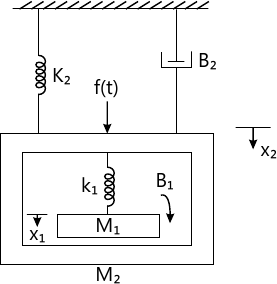6 M
1 (c) Obtain the transfer function of an armature controlled DC servomotor.
6 M

2 (a) Obtain the transfer function of the block diagram shown below, using block diagram reduction technique.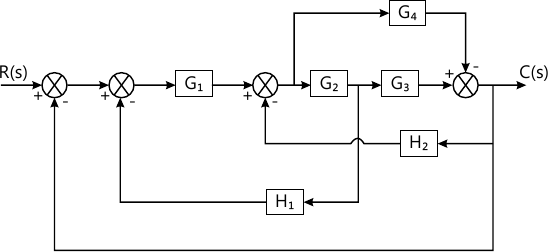10 M
2 (b) Obtain the closed loop transfer function C(s)/R(s) for the signal flow graph of a system shown in Fig. Q2(b) by use of Mason's gain formula.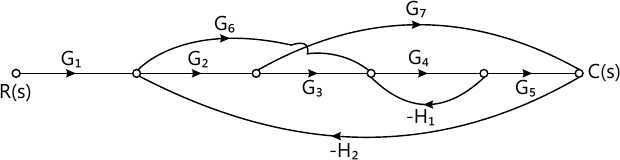10 M

3 (a) Derive expression for peak response time tp and maximum overshoot Mp of an under damped second order control system subjected to step input.
6 M
3 (b) A second order control system is represented by a transfer function given below: $\dfrac {0_0(s)}{T(s)}= \dfrac {1}{Js^2+Fs+K}$ where ?0 is the proportional output and T is the input torque. A step unit of 10 N-m is applied to the system and test results are given below:
i) Maximum overshoot is 6%
ii) Peak time is 1 sec
Determine the values of J, F and K.
8 M
3 (c) For a unity feedback control system with $G(s)= \dfrac {10(s+2)}{s^2 (s+1)}$ find:
i) The static error coefficients
ii) Steady state error when the input transform is $R(s)= \dfrac {3}{s}- \dfrac {2}{s^2}- \dfrac {1}{3s^2}$
6 M

4 (a) Explain Routh-Hurwitz's criterion for determining the stability of a system and mention any three limitations of R-H criterion.
10 M
4 (b) A unity feedback control system is characterized by the open loop transfer function: $G(s) = \dfrac {K(s+13)} {s(s+3)(s+7)}$ i) Using the Routh's criterion, calculate the range of values of K for the system to be stable.
ii) Check if for K=1, all the roots of the characteristic equation of the above system are more negative than -0.5.
10 M

5 (a) Sketch the root locus for a unity feedback control system with open loop transfer function: $G(s)= \dfrac {K(s+2)(s+3)}{s(s+1)}$
12 M
5 (b) Show that the root Loci for unity feedback control system with $G(s)=\dfrac {K(s^2 +6s +10)}{(s^2+2s+10)}$ are the areas of circle of radius √10 and centred at the origin.
8 M

6 (a) Sketch the Bode plot of a unity feedback system whose open loop transfer function is given by $G(s)= \dfrac {K}{s(1+0.1s)(s+0.05s)}$ i) Find the value of K for a gain margin of 10dB.
ii) Find the value of K for a phase margin of 30°.
14 M
6 (b) Determine the open loop transfer function of a system whose approximate plot is shown below.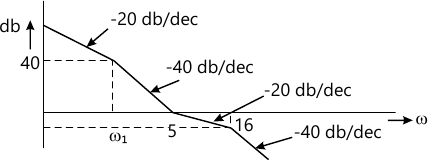6 M

7 (a) State and explain Nyquist stability criterion.
6 M
7 (b) Sketch the Nyquist plot for a system whose open loop transfer function is $G(s)H(s)= \dfrac {K(4s+1)}{s(2s-1)}$ Determine the range of K for which the system is stable.
14 M

8 (a) Define state variable and state transition matrix. List the properties of the state transition matrix.
8 M
8 (b) Obtain the state model of the electrical network shown in below, by choosing V1(t) and V2(t) as state variables.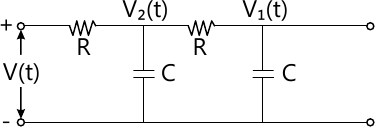12 M

More question papers from Control Systems